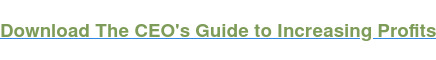# Cost Volume Profit Formula

Cost-Volume-Profit (CVP) Model

# Cost Volume Profit Formula: Breakeven Sales Volume

Breakeven Sales Volume = Fixed Costs ÷ (Sales Price – Variable Costs)

6,000 = \$30,000 ÷ (\$7 – \$2)

6,000 = \$30,000 ÷ (\$5)

As you can see, the theater has a contribution margin of \$5. That is, the theater makes five dollars per ticket sold. This contribution margin can be used to pay down the theater’s fixed costs. So we divide \$30,000 of fixed costs by \$5 contribution margin. This shows us that the theater must sell 6,000 tickets per quarter to break even. The cost volume profit equation shows us many important aspects of the business of the theater.

Now let’s say the theater doesn’t want to merely breakeven. They actually want to make a profit in the upcoming quarter. Selling 6,000 tickets allows them to breakeven. But how many do they need to sell in order to make a profit of, let’s say, \$10,000? We can find out by using the CVP model and the CVP formula.

When performing CVP analysis in order to determine the sales volume required for a set target profit, you simply add the target profit to the fixed costs. So we have variable costs of \$2, sales price of \$7, and fixed costs of \$30,000. And now we’re adding target profit of \$10,000. Following is how we set up the CVP formula for a target profit.## Cost Volume Profit Calculation: Target Sales Volume

Target Sales Volume = (Fixed Costs + Target Profit) ÷ (Sales Price – Variable Costs)

Target Sales Volume = (Fixed Costs + Target Profit) ÷ (Contribution Margin)

8,000 = (\$30,000 + \$10,000) ÷ (\$7 – \$2)

8,000 = (\$40,000) ÷ (\$5)

As you see here, the theater must sell 8,000 tickets in order to cover its fixed costs and make a profit of \$10,000 in the upcoming quarter.

Of course, for illustrative purposes, this is a very simple example. Real-world examples may be more complex and have more variables. But this is a basic version of the cost-volume-profit financial model.